1592687400

# Subjectivity Classification with Convolutional Neural Networks

Natural language processing (NLP) is a subfield of linguistics and artificial intelligence concerned with the interactions between computers and human (natural) languages. The ultimate objective of NLP is to read, decipher, understand, and make sense of the human languages in a manner that is valuable.
In this post we the process of creating a deep learning model from scratch in PyTorch. We implement the approach described in this paper for classifying sentences using Convolutional Neural Networks (CNNs).

#machine-learning #convolutional-network #pytorch #deep-learning #python

## Buddha Community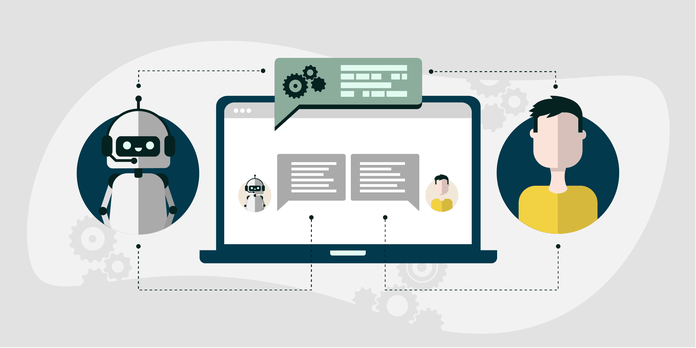1598554500

## Satellite image classification with a convolutional neural network.

My latest project at Flatiron was to use neural networks to classify satellite image tiles. I chose to use a convolutional neural network (CNN) and create a dataset of webscraped images to train the model with. This will just be a quick rundown of what went into the project with additional links to my articles to more of the technical parts. This way, it can help to familiarize you with the topics or help to share more about my work with those who have similar interests in computer vision and machine learning.

I chose to use a CNN because I read some school lessons on computer vision about how a CNN has advantages with image classification. A CNN uses pooling layers that filter through patches of the image pixels, finding common patterns, which develop into more complex patterns in order to help determine image class. I chose to work on a computer vision project with satellite images because there are possible use cases for solutions on Earth as well as use cases on other planets. I’ve read articles about organizations looking at different geological patterns on the Mars surface in search of the possible presence of water or perhaps its pre-existence on the planet. This led me to try and build the model to recognize river delta patterns here on Earth, with the next step being to train the model and locate delta patterns on Mars. The model could also eventually be useful for looking at changes to river deltas on Earth, for possible use in agriculture, climate change or even real estate. For now, the project is ongoing as of my writing this blog post, with training and testing performed on the Earth images. The Mars images will be the next part I’ll begin after graduation.

A land image tile.

A river delta image tile.

A Mars delta image tile.

In order to obtain the images for a dataset, I looked into some different API’s and webscraping with Beautiful Soup. Afterwards, I decided to use Selenium to scrape some images from an image search on Google. This method was able to scroll through the page interactively, which was necessary in order to have access to all of the images. I wrote a separate article about that process here. This method was useful as a starting point, in order to go through the process of building the dataset, creating the model, training, testing and just getting everything to work. The disadvantage was that there were a lot of images that were not clean, contained pieces of text or other image artifacts and overall led to less accurate results. There are some example images below so you can see what I mean. I do not claim copyright to any of the used images as they were used for an educational project for school and will remove them if anyone objects to their display on my article.

#convolutional-network #computer-vision #python #image-classification #machine-learning #neural networks1596826500

## Convolutional Neural Networks-An Intuitive approach

A simple yet comprehensive approach to the concepts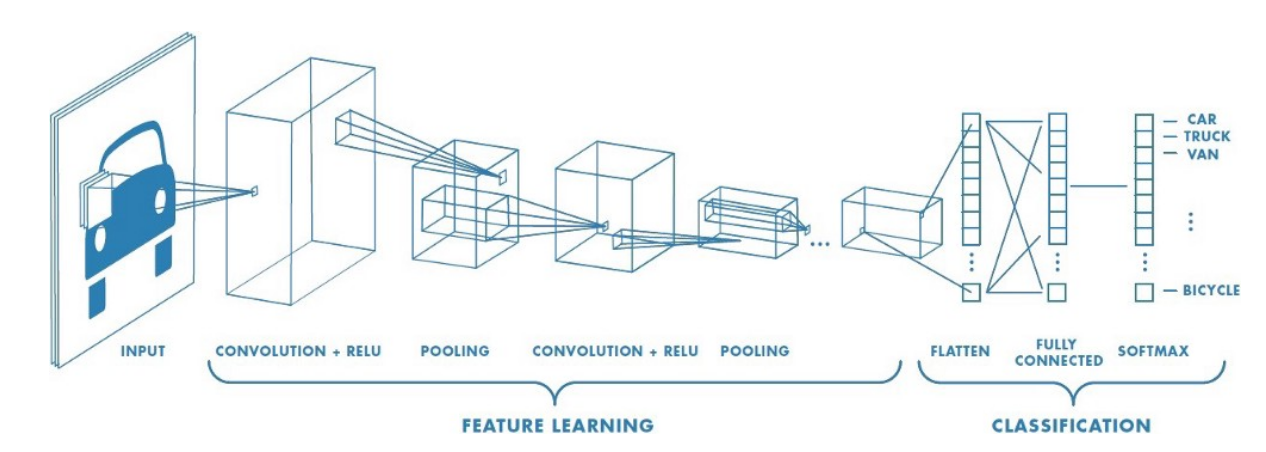Convolutional Neural Networks

Artificial intelligence has seen a tremendous growth over the last few years, The gap between machines and humans is slowly but steadily decreasing. One important difference between humans and machines is (or rather was!) with regards to human’s perception of images and sound.How do we train a machine to recognize images and sound as we do?

At this point we can ask ourselves a few questions!!!

How would the machines perceive images and sound ?

How would the machines be able to differentiate between different images for example say between a cat and a dog?

Can machines identify and differentiate between different human beings for example lets say differentiate a male from a female or identify Leonardo Di Caprio or Brad Pitt by just feeding their images to it?

Let’s attempt to find out!!!

The Colour coding system:

Lets get a basic idea of what the colour coding system for machines is

RGB decimal system: It is denoted as rgb(255, 0, 0). It consists of three channels representing RED , BLUE and GREEN respectively . RGB defines how much red, green or blue value you’d like to have displayed in a decimal value somewhere between 0, which is no representation of the color, and 255, the highest possible concentration of the color. So, in the example rgb(255, 0, 0), we’d get a very bright red. If we wanted all green, our RGB would be rgb(0, 255, 0). For a simple blue, it would be rgb(0, 0, 255).As we know all colours can be obtained as a combination of Red , Green and Blue , we can obtain the coding for any colour we want.

Gray scale: Gray scale consists of just 1 channel (0 to 255)with 0 representing black and 255 representing white. The colors in between represent the different shades of Gray.

Computers ‘see’ in a different way than we do. Their world consists of only numbers.

Every image can be represented as 2-dimensional arrays of numbers, known as pixels.

But the fact that they perceive images in a different way, doesn’t mean we can’t train them to recognize patterns, like we do. We just have to think of what an image is in a different way.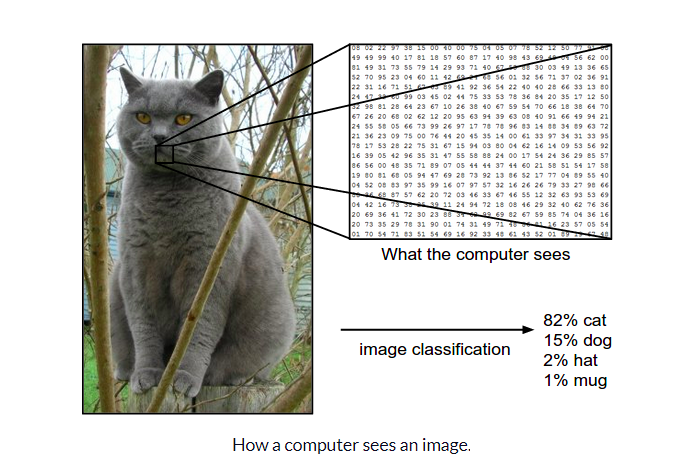Now that we have a basic idea of how images can be represented , let us try and understand The architecture of a CNN

CNN architecture

Convolutional Neural Networks have a different architecture than regular Neural Networks. Regular Neural Networks transform an input by putting it through a series of hidden layers. Every layer is made up of a set of neurons, where each layer is fully connected to all neurons in the layer before. Finally, there is a last fully-connected layer — the output layer — that represent the predictions.

Convolutional Neural Networks are a bit different. First of all, the layers are organised in 3 dimensions: width, height and depth. Further, the neurons in one layer do not connect to all the neurons in the next layer but only to a small region of it. Lastly, the final output will be reduced to a single vector of probability scores, organized along the depth dimension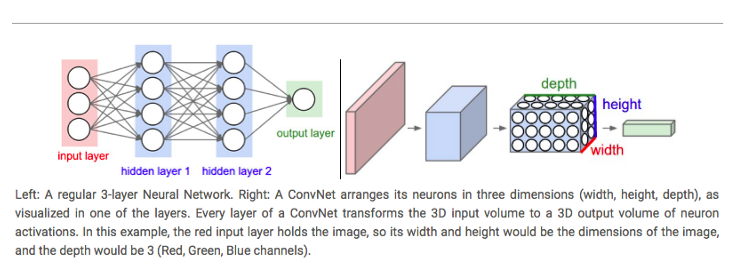A typical CNN architecture

As can be seen above CNNs have two components:

• The Hidden layers/Feature extraction part

In this part, the network will perform a series of **convolutions **and pooling operations during which the features are detected. If you had a picture of a tiger , this is the part where the network would recognize the stripes , 4 legs , 2 eyes , one nose , distinctive orange colour etc.

• The Classification part

Here, the fully connected layers will serve as a classifier on top of these extracted features. They will assign a** probability** for the object on the image being what the algorithm predicts it is.

Before we proceed any further we need to understand what is “convolution”, we will come back to the architecture later:

What do we mean by the “convolution” in Convolutional Neural Networks?

Let us decode!!!

#convolutional-neural-net #convolution #computer-vision #neural networks1596825840

## A Comparative Analysis of Recurrent Neural Networks

Recurrent neural networks, also known as RNNs, are a class of neural networks that allow previous outputs to be used as inputs while having hidden states. RNN models are mostly used in the fields of natural language processing and speech recognition.

The vanishing and exploding gradient phenomena are often encountered in the context of RNNs. The reason why they happen is that it is difficult to capture long term dependencies because of multiplicative gradient that can be exponentially decreasing/increasing with respect to the number of layers.

Gated Recurrent Unit (GRU) and Long Short-Term Memory units (LSTM) deal with the vanishing gradient problem encountered by traditional RNNs, with LSTM being a generalization of GRU.

1D Convolution_ layer_ creates a convolution kernel that is convolved with the layer input over a single spatial (or temporal) dimension to produce a tensor of outputs. It is very effective for deriving features from a fixed-length segment of the overall dataset. A 1D CNN works well for natural language processing (NLP).

## DATASET: IMDb Movie Review

TensorFlow Datasets is a collection of datasets ready to use, with TensorFlow or other Python ML frameworks, such as Jax. All datasets are exposed as `[_tf.data.Datasets_](https://www.tensorflow.org/api_docs/python/tf/data/Dataset)`, enabling easy-to-use and high-performance input pipelines.

“imdb_reviews”

This is a dataset for binary sentiment classification containing substantially more data than previous benchmark datasets. It provides a set of 25,000 highly polar movie reviews for training, and 25,000 for testing.

## Import Libraries

``````import pandas as pd
import numpy as np
import matplotlib.pyplot as plt
import seaborn as sns
%matplotlib inline
``````

``````import tensorflow as tf
import tensorflow_datasets

imdb
``````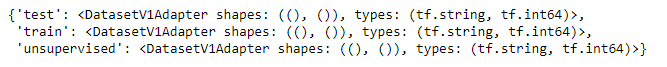``````info
``````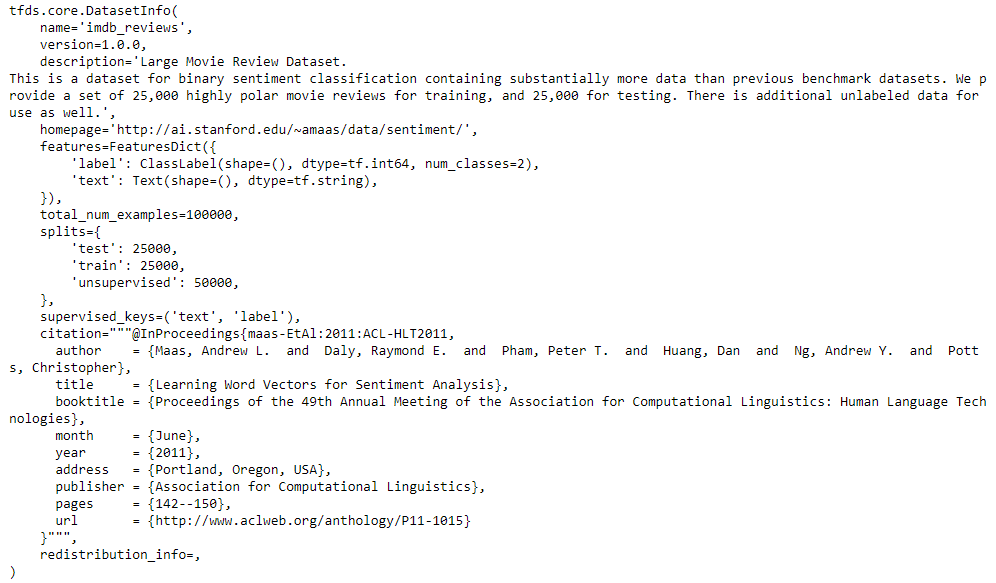## Training and Testing Data

``````train_data, test_data=imdb['train'], imdb['test']

training_sentences=[]
training_label=[]
testing_sentences=[]
testing_label=[]
for s,l in train_data:
training_sentences.append(str(s.numpy()))
training_label.append(l.numpy())
for s,l in test_data:
testing_sentences.append(str(s.numpy()))
testing_label.append(l.numpy())
training_label_final=np.array(training_label)
testing_label_final=np.array(testing_label)
``````

``````vocab_size=10000
embedding_dim=16
max_length=120
trunc_type='post'
oov_tok='<oov>'
from tensorflow.keras.preprocessing.text import Tokenizer
tokenizer= Tokenizer(num_words=vocab_size, oov_token=oov_tok)
tokenizer.fit_on_texts(training_sentences)
word_index=tokenizer.word_index
sequences=tokenizer.texts_to_sequences(training_sentences)
testing_sequences=tokenizer.texts_to_sequences(testing_sentences)
from tensorflow.keras.models import Sequential
from tensorflow.keras.layers import Dense, Dropout, Embedding
``````

## Multi-layer Bidirectional LSTM

#imdb #convolutional-network #long-short-term-memory #recurrent-neural-network #gated-recurrent-unit #neural networks1594935240

## Convolutional Neural Networks(CNN’s) — A practical perspective

Hello everyone,

Hoping that everyone is safe and doing well. In this blog, we will look into some concepts of CNN’s for image classification that are often missed or misunderstood by beginners (including me till some time back). This blog requires the reader to have some basic idea of how CNN’s work. However, we will cover the important aspects of CNN’s before getting deeper into advanced topics.

After this, we will look at a machine learning technique called Transfer learning and how it is useful in training a model with less data on a deep learning framework. We will train an image classification model on top of Resnet34 architecture using the data that contains digitally recorded heartbeats of human beings in the form of audio (.wav) files. In the process, we will convert each of these audio files into an image by converting them to spectrograms using a popular python audio library called Librosa. In the end, we will examine the model with popular error metrics and check its performance.

## CNN’s for image classification:

Neural networks with more than 1 convolution operations are called convolutional neural networks (CNN). Input of a CNN contains images with numerical values in each pixel arranged spatially along the width, height and depth (channels). The goal of the total architecture is to get a probability score of an image belonging to a certain class by learning from these numerical values arranged spatially. In the process, we perform operations like pooling and convolutions on these numerical values to squeeze and stretch them along the depth.

An image typically contains three layers namely RGB (Red, Green, Blue).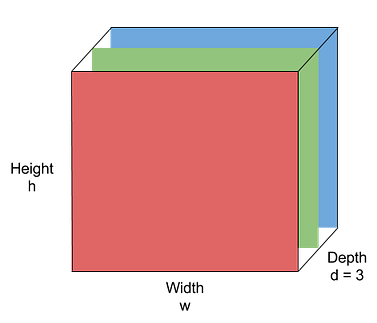Image by Purit Punyawiwat, Source : Datawow

## Main operations in CNN’s

Convolution operation

Convolution operation is (w.x+b) applied to all the different spatial localities in the input volume. Using more number of convolution operations helps to learn a particular shape even if its location in the image is changed.

Example: Generally clouds are present on the top of a landscape image. If an inverted image is fed into a CNN, more number of convolutional operations makes sure that the model identifies the cloud portion even if it is inverted.

Mathematical Expression: x_new = w.x + b where w is the filter/kernel, b is the bias and x is part of a hidden layer output. Both w and  _b _are different for every convolution operation applied on different hidden layers.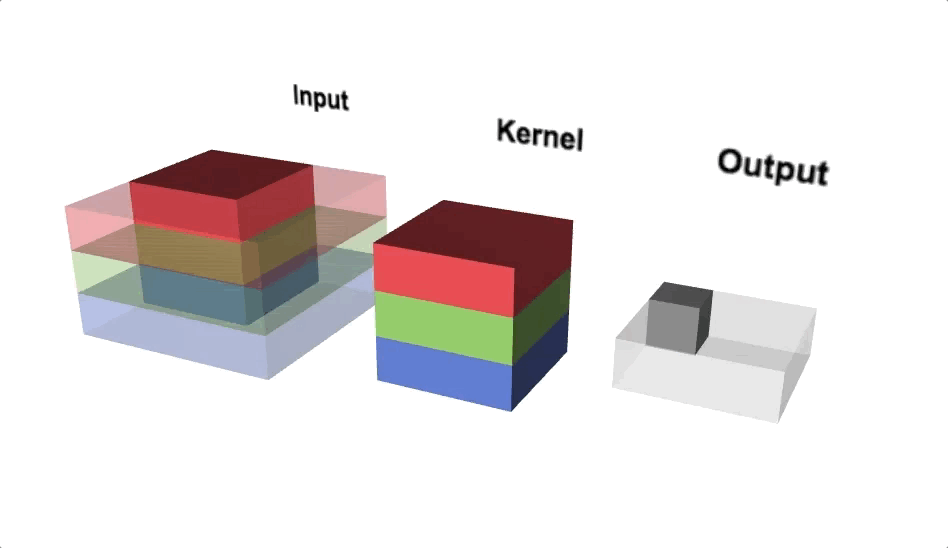Convolution Operations(Source: Lecture 22-EECS251, inst.eecs.berkley.edu)

Pooling

Pooling reduces the spatial dimensions of each activation map (output after convolution operation) while aggregating the localised spatial information. Pooling helps to squeeze the output from hidden layers along height and width. If we consider maximum value within the non-overlapping sub-regions then it is called Max-pooling. Max-pooling also adds non-linearity to the model.

#pytorch #deep-learning #transfer-learning #audio-classification #convolutional-network #neural networks1595691960

## How Graph Convolutional Networks (GCN) Work

In this post, we’re gonna take a close look at one of the well-known Graph neural networks named GCN. First, we’ll get the intuition to see how it works, then we’ll go deeper into the maths behind it.

## Why Graphs?

Many problems are graphs in true nature. In our world, we see many data are graphs, such as molecules, social networks, and paper citations networks.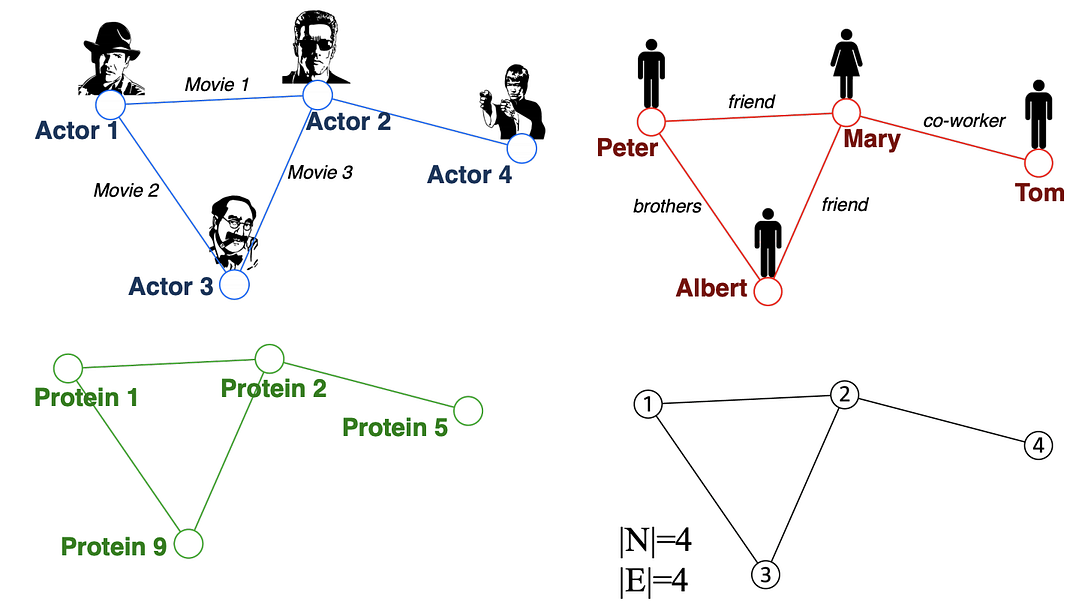Examples of graphs. (Picture from )

• Node classification: Predict a type of a given node
• Community detection: Identify densely linked clusters of nodes
• Network similarity: How similar are two (sub)networks

## Machine Learning Lifecycle

In the graph, we have node features (the data of nodes) and the structure of the graph (how nodes are connected).

For the former, we can easily get the data from each node. But when it comes to the structure, it is not trivial to extract useful information from it. For example, if 2 nodes are close to one another, should we treat them differently to other pairs? How about high and low degree nodes? In fact, each specific task can consume a lot of time and effort just for Feature Engineering, i.e., to distill the structure into our features.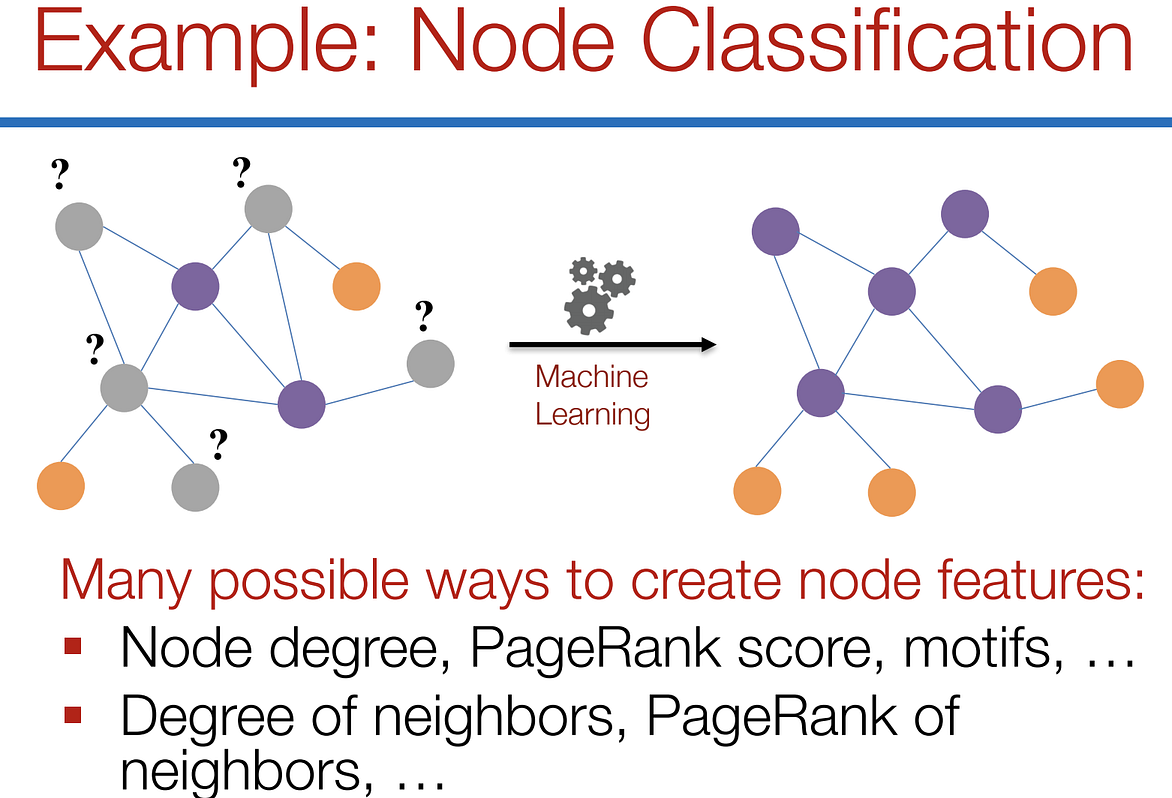Feature engineering on graphs. (Picture from )

It would be much better to somehow get both the node features and the structure as the input, and let the machine to figure out what information is useful by itself.

That’s why we need Graph Representation Learning.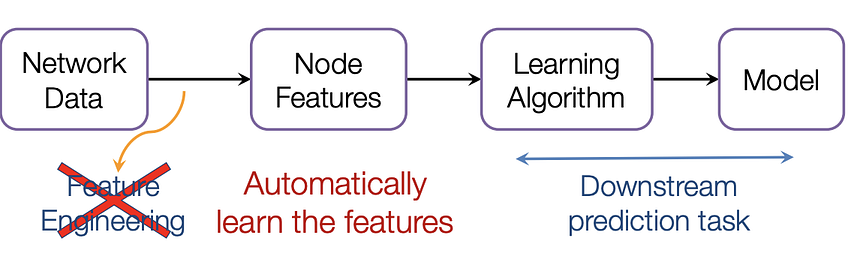We want the graph can learn the “feature engineering” by itself. (Picture from )

# Graph Convolutional Networks (GCNs)

Paper: Semi-supervised Classification with Graph Convolutional Networks(2017) 

GCN is a type of convolutional neural network that can work directly on graphs and take advantage of their structural information.

it solves the problem of classifying nodes (such as documents) in a graph (such as a citation network), where labels are only available for a small subset of nodes (semi-supervised learning).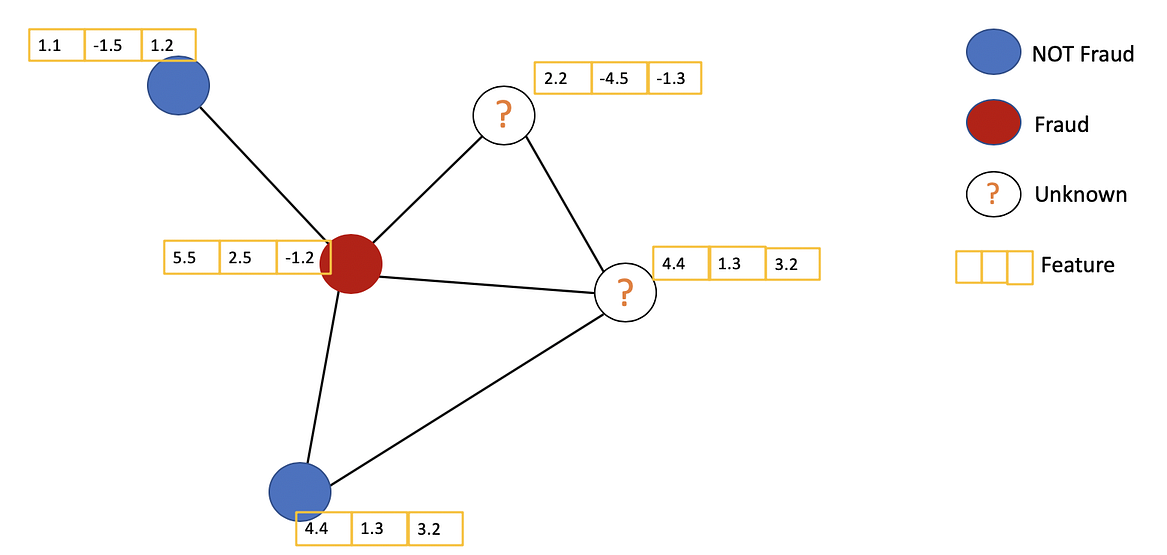Example of Semi-supervised learning on Graphs. Some nodes dont have labels (unknown nodes).

#graph-neural-networks #graph-convolution-network #deep-learning #neural-networks